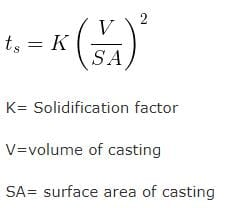# Test: Production Engineering- 1

## 25 Questions MCQ Test Mechanical Engineering SSC JE (Technical) | Test: Production Engineering- 1

Description
Attempt Test: Production Engineering- 1 | 25 questions in 50 minutes | Mock test for Mechanical Engineering preparation | Free important questions MCQ to study Mechanical Engineering SSC JE (Technical) for Mechanical Engineering Exam | Download free PDF with solutions
QUESTION: 1

Solution:
QUESTION: 2

Solution:
QUESTION: 3

### Which of the following is a point imperfection; 1. vacency 2. frenkel defect 3. interstitialcy 4. Schottky defect

Solution:
QUESTION: 4

Isothermal annealing is mainly used in alloy steel to improne.

Solution:
QUESTION: 5

The process of reheating to reduce brittleness without any significant loss in hardness is

Solution:
QUESTION: 6

The main purpose of chaplets is

Solution:
QUESTION: 7

Green sand mould indicates that

Solution:
QUESTION: 8

Solution:
QUESTION: 9

The gating ratio 2 : 8 : 1. for copper in gating system refers to the ratio of arae of

Solution:
QUESTION: 10

Two streams of liquid metal which are not hot enough to fuse properly result into a casting defect known as :-

Solution:
QUESTION: 11

Shell moulding can be used for.

Solution:
QUESTION: 12

An expandable pattern is used in

Solution:
QUESTION: 13

In which of the following metal moulds are used

Solution:
QUESTION: 14

Which of the following is true for centrifugal casting

Solution:
QUESTION: 15

If the diameter of the hole is subject to considerable variation, then for locating in jigs and fixtures, the pressure type of locator used is

Solution:
QUESTION: 16

The major difficulty during welding of aluminium is

Solution:
QUESTION: 17

The ratio of acetelene to oxygen is approximately _______ for a neutral flame used in gas welding.

Solution:
QUESTION: 18

In oxy acetelene gas welding the temperature at the inner core of flame is about

Solution:
QUESTION: 19

In are welding, penetration is minimum for

Solution:
QUESTION: 20

The coating material of an arc welding electrode contains which of the following
1. Deoxidising agent
2. Arc stabilizing agent
3. Slag forming agent

Solution:
QUESTION: 21

Arc Blow is more common in

Solution:
QUESTION: 22

In an inert gas welding, the commonly used gas is

Solution:
QUESTION: 23

Which of the following are welding process does not used consumable electrodes.

Solution:
QUESTION: 24

In resistance welding, heat is produced due to resistance between

Solution:
QUESTION: 25

According to Chvorinov’s equation, the solidification time of a casting is proportional to (V is volume of casting):

Solution:Use Code STAYHOME200 and get INR 200 additional OFF Use Coupon Code